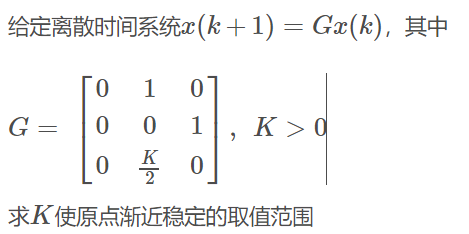# 第12周：李雅普诺夫稳定性（2）--2. 离散系统的稳定性【含答案】 自动控制理论

（1）单选题

A  正确

B  错误

（2）单选题A  $$K>4$$

B  $$0< K <4$$

C  $$0< K <2$$

D  $$K>2$$